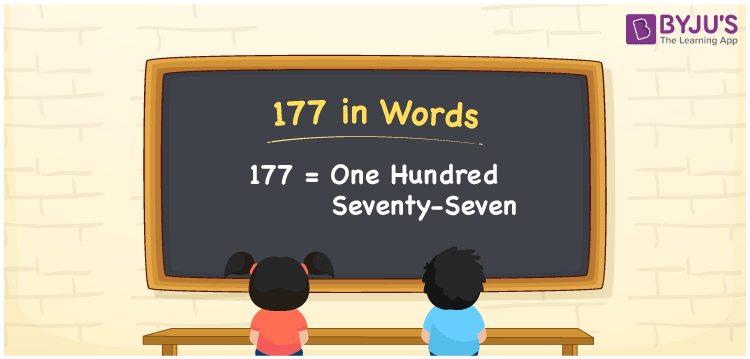# 177 in Words

We can write 177 in words as One hundred seventy-seven. If you have enlisted 177 students’ names in an attendance register, you can say, “I enlisted One hundred seventy-seven students’ names in the attendance register”. Let’s learn how to convert the cardinal number 177 to a word form here in this article, along with some facts about 177.

 177 in words One hundred seventy-seven One hundred seventy-seven in Numbers 177

## 177 in English Words

Generally, we use the English alphabet to write numbers in words. Thus, we spell 177 in English words as “One hundred seventy-seven”.## How to Write 177 in Words?

As we can see, 177 is a three-digit number which means, we need to create a place value chart with three columns as given below.

 Hundreds Tens Ones 1 7 7

Here, ones = 7, tens = 7, hundreds = 1

By expanding these numbers with respect to place values, we get;

1 × Hundred + 7 × Ten + 7 × One

= 100 + 70 + 7

= One hundred + Seventy + Seven

= One hundred seventy-seven

Therefore, 177 in words = One hundred seventy-seven

As we know, 177 is a natural number that precedes 178 and succeeds 176.

177 in words – One hundred seventy-seven

Is 177 an even number? – No

Is 177 an odd number? – Yes

Is 177 a prime number? – No

Is 177 a composite number? – Yes

Is 177 a perfect square number? – No

Is 177 a perfect cube number? – No

## Frequently Asked Questions on 177 in Words

### How do you write 177 in words?

We can write the number 177 in words as One hundred seventy-seven.

### Write the value of 1 + 177 in words.

1 + 177 = 178 Thus, the value of 1 + 177, i.e., 178 can be written in words as One hundred seventy-eight.

### How to write Rs. 177 in words on a cheque?

On a cheque, we generally write Rs. 177 in words as “One hundred seventy-seven rupees only”.